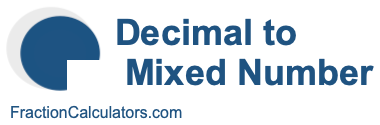What is 5.751 as a mixed number?Here we will show you how to convert the decimal number 5.751 to a mixed number. In other words, we will show you how to convert 5.751 to a whole number (W) and a fraction with a numerator (N) and a denominator (D). Here is the illustration of what we are going to convert:

5.751 ->> Mixed Number
5.751 ->> W N/D

Before we continue, and in case you are wondering, note that mixed number is the same as mixed fraction. Thus, 5.751 as a mixed number is the same as 5.751 as a mixed fraction. Anyway, here are step-by-step instructions showing you how to get 5.751 as a mixed number:

The whole number is the number to the left of the decimal point. Therefore, the whole number (W) is equal to 5.

The numerator is the number to the right of the decimal point. Therefore, the numerator (N) is 751.

The get the denominator, you take 10 to the power of the number of digits in the numerator. There are three digits in the numerator and 10^3 is 1000, which means that the denominator is 1000.

When we put it all together and simplify if necessary, we get the answer to 5.751 as a mixed number, like this:

5.751 = 5 751/1000
5.751 = 5 751/1000
5.751 as a mixed number = 5 751/1000

Decimal to Mixed Number Calculator
Use this tool to convert another decimal number to a mixed number.

What is  as a mixed number?

What is 5.752 as a mixed number?
Here is another decimal number that we have converted to a mixed number for you.